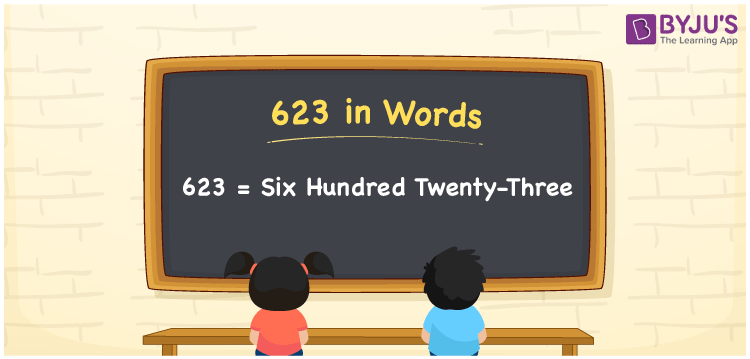# 623 in Words

623 in words is written as Six hundred twenty-three. In both the International System of Numerals and the Indian System of Numerals, 623 is written as Six hundred twenty-three. The number 623 is a Cardinal Number as it represents some quantity. For example, “623 students have participated in the test”.

 623 in Words Six hundred twenty-three Six hundred twenty-three in Number 623

## 623 in English Words

We write 623 in English Words using the letters of the English alphabet. Therefore, we read 623 in English as “Six hundred twenty-three.”## How to Write 623 in Words?

To write 623 in words, we shall use the place value chart. In the place value chart, write 6 in the hundreds, 2 in the tens, and 3 in the ones, respectively. Now let us make a place value chart to write the number 623 in words.

 Hundreds Tens Ones 6 2 3

Thus, we can write the expanded form as

6 × Hundred + 2 × Ten + 3× One

= 6 × 100 + 2 × 10 + 3× 1

= 600 + 20+ 3

= 623

= Six hundred twenty-three.

623 is a natural number, the successor of 622 and the predecessor of 624.

623 in words – Six hundred twenty-three

• Is 623 an odd number? – Yes
• Is 623 an even number? – No
• Is 623 a perfect square number? – No
• Is 623 a perfect cube number? – No
• Is 623 a prime number? – No
• Is 623 a composite number? – Yes

## Frequently Asked Questions on 623 in Words

Q1

### How to write 623 in words?

623 in words is written as Six hundred twenty-three.
Q2

### How to write 623 in the International and Indian System of Numerals?

In both, the system of numerals, 623 in words, is written as Six hundred twenty-three.
Q3

### What is the preceding number of 623?

The number that precedes 623 is 622.